Question

# Write a JavaScript program to calculate Area of a trapezoid Equation: Area = (top side +...

Write a JavaScript program to calculate Area of a trapezoid
Equation: Area = (top side + bottom side) * height / 2
1. Use prompt() function to take top side, bottom side, and height values from user
2. For validation, if user did not put in a number, your program must send out
3. "Input must be number" error message using alert() function.
4. If user put in all numbers, use alert() function to output correct answer

Java Script program:

var top = parseInt(prompt("Enter top side of Trapazoid : "));

var bottom = parseInt(prompt("Enter bottom side of Trapazoid : "));

var height = parseInt(prompt("Enter height of Trapazoid : "));

var area = (top+bottom)*height/2;

if(isNaN(top)|| isNaN(bottom)||isNaN(height))

else

alert("The area of trapazoid is "+area);

#### Earn Coins

Coins can be redeemed for fabulous gifts.

Similar Homework Help Questions
• ### Write a JavaScript program to calculate Area of a trapezoid Equation: Area = (top side +...

Write a JavaScript program to calculate Area of a trapezoid Equation: Area = (top side + bottom side) * height / 2 1. Use prompt() function to take top side, bottom side, and height values from user 2. For validation, if user did not put in a number, your program must send out 3. "Input must be number" error message using alert() function. 4. If user put in all numbers, use alert() function to output correct answer

• ### Question 4 Write a program that display the area of a triangle. The program calls the...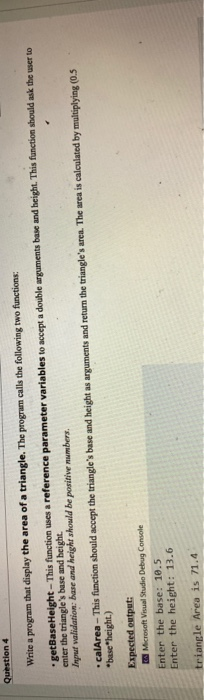Question 4 Write a program that display the area of a triangle. The program calls the following two functions: .getBaseHeight - This function uses a reference parameter variables to accept a double arguments base and height. This function should ask the user to enter the triangle's buse and height. Input validation: base and height should be positive numbers. calArea - This function should accept the triangle's base and height as arguments and return the triangle's area. The area is calculated...

• ### Programing requirement for: Safety Alert System Version 01 I. Project and class name Create a new...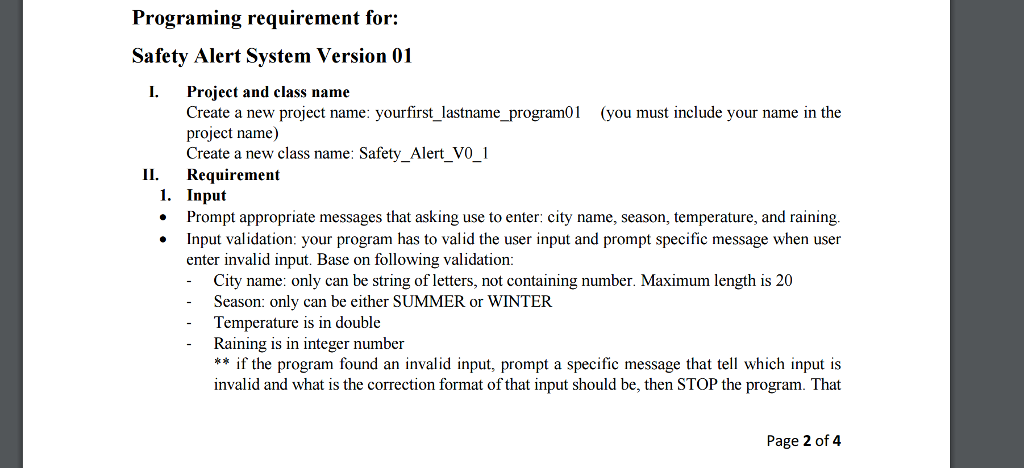Programing requirement for: Safety Alert System Version 01 I. Project and class name Create a new project name: yourfirst lastname program01 (you must include your name in the project name) Create a new class name: Safety Alert vo 1 II. Requirement 1. Input Prompt appropriate messages that asking use toenter: city name, season, temperature, and raining. Input validation: your program has to valid the user input and prompt specific message when user enter invalid input. Base on following validation: City...

• ### Write a complete program for Visual C++ to calculate and display the volume of a cylinder, given...

Write a complete program for Visual C++ to calculate and display the volume of a cylinder, given its radius and height. Your program must meet the followingrequirements:· Your program should prompt the user to input the radius and height. The program should output the result with three digits after the decimal point. The output shouldinclude some explanatory text.· Make the value of PI (3.14159) a constant in your program.· The volume formula is PI * radius2 * height.· Valid input...

• ### 1. Prompt the user for one of the arithmetic operators ('op'): +, -, *,/, or **...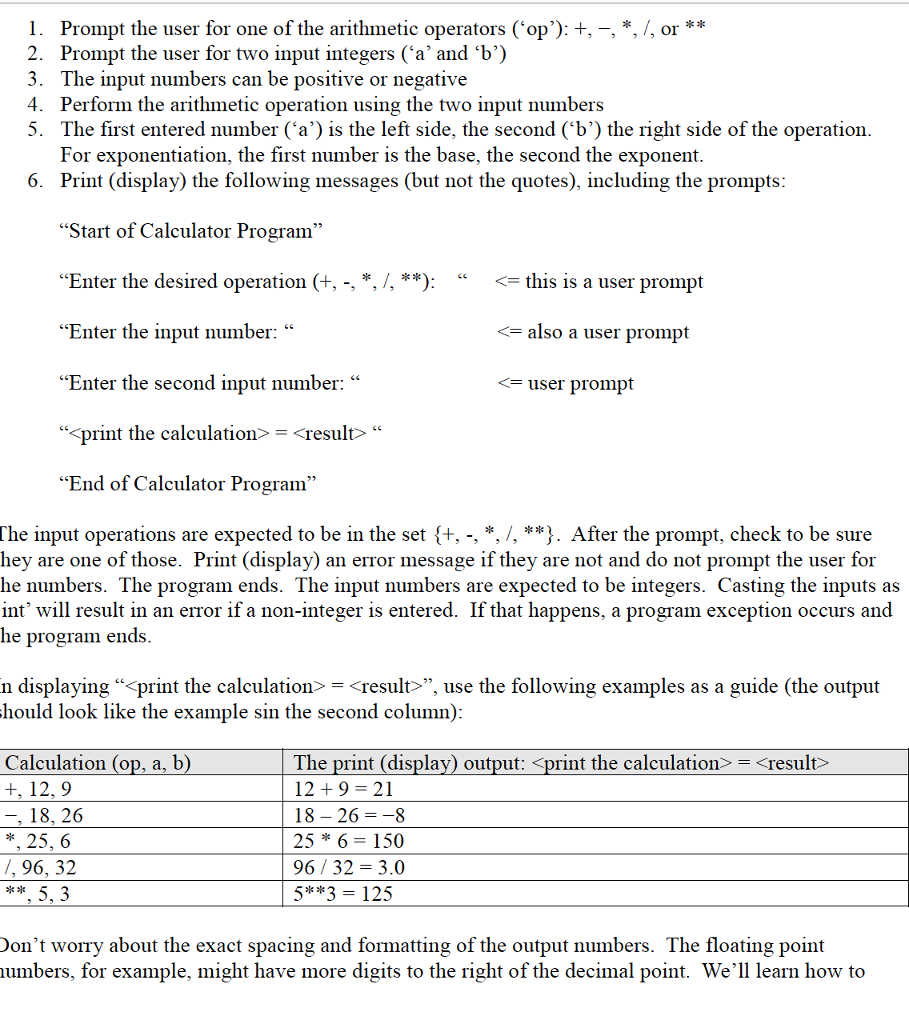1. Prompt the user for one of the arithmetic operators ('op'): +, -, *,/, or ** 2. Prompt the user for two input integers ('a' and'b') 3. The input numbers can be positive or negative 4. Perform the arithmetic operation using the two input numbers 5. The first entered number ('a') is the left side, the second ('b') the right side of the operation For exponentiation, the first number is the base, the second the exponent Print (display) the following...

• ### Using C++, Write a program that will provide the user a menu from which the user...

Using C++, Write a program that will provide the user a menu from which the user may select to calculate the area of one of four geometric shapes: a circle, a rectangle, a triangle, and a trapezoid. You should use the most appropriate SWITCH block for this program. The user will input all data needed to calculate the area. The program should output all data input by the user, the calculated area, and the geometric shape selected. Run this program...

• ### Write a program **(IN C)** that displays all the phone numbers in a file that match the area code...

Write a program **(IN C)** that displays all the phone numbers in a file that match the area code that the user is searching for. The program prompts the user to enter the phone number and the name of a file. The program writes the matching phone numbers to the output file. For example, Enter the file name: phone_numbers.txt Enter the area code: 813 Output: encoded words are written to file: 813_phone_numbers.txt The program reads the content of the file...

• ### IN JAVASCRIPT 5. Write the pseudo code to solve the following problem (10pts/10pts) You are asked...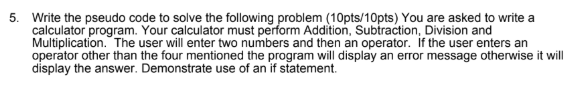IN JAVASCRIPT 5. Write the pseudo code to solve the following problem (10pts/10pts) You are asked to write a calculator program. Your calculator must perform Addition, Subtraction, Division and Multiplication. The user will enter two numbers and then an operator. If the user enters an operator other than the four mentioned the program will display an error message otherwise it will display the answer. Demonstrate use of an if statement

• ### Use C programming Make sure everything works well only upload Write a program that takes an...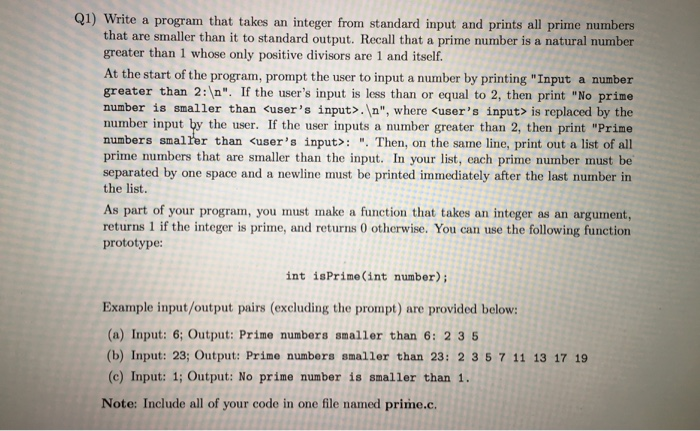Use C programming Make sure everything works well only upload Write a program that takes an integer from standard input and prints all prime numbers that are smaller than it to standard output. Recall that a prime number is a natural number greater than 1 whose only positive divisors are 1 and itself. At the start of the program, prompt the user to input a number by printing "Input a number greater than 2:\n". If the user's input is less...

• ### Part A) Write a C++ program that calculates the area under a curve. Here is the...

Part A) Write a C++ program that calculates the area under a curve. Here is the equation: f(x) = x^2 +1.5x +4 You must prompt the user for the beginning and the ending x values. You are to assume, but not check, that the user will put in whole positive numbers. The units are inches. The program input/output should look something like this: This program calculates the area under a curve between two points on the x axis. The equation...